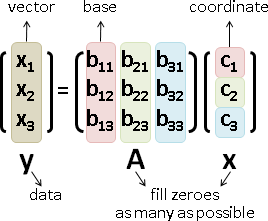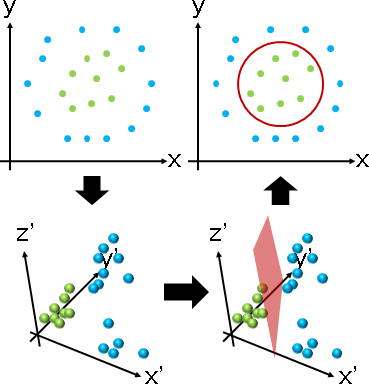Representation

Representation

「重新表示」。換個視角看世界。數據實施變換，改變姿態。```變換形式：已知原數據，求新數據、求函數。

``````線性組合：直條的加權平均。（圖例是分解形式）

``````左乘：數據們實施線性變換。原數據與新數據一一對應。（圖例是變換形式）

```

```min ‖y - Ax‖²          向量的重新表示y = Ax（此例是分解形式）
A,x
```
```min ‖Y - AX‖F²         矩陣的重新表示Y = AX（此例是分解形式）
A,X
```

Sparse Representation

Sparse Representation

```min ‖y - Ax‖F² + α ‖A‖₀ + β ‖x‖₀     (α ≥ 0 and β ≥ 0)
A,x
```《Online Dictionary Learning for Sparse Coding》

Sparse Matrix Factorization

```min ‖M - AB‖F² + α ‖A‖₀ + β ‖B‖₀     (α ≥ 0 and β ≥ 0)
A,B
```

Factorization Machine

Sparse Principal Component Analysis

PCA的數學式子有兩種等價寫法，這裡採用第二種。

```max ‖YᵀX‖F²   subject to YᵀY = I
Y
```

```min ‖X - YYᵀX‖F²   subject to YᵀY = I
Y
```

```min ‖X - YYᵀX‖F² + α (‖Y‖F² - n)     (α ≥ 0)
Y
min ‖X - YYᵀX‖F² + α ‖Y‖F²           (α ≥ 0)
Y
```

```min ‖X - YYᵀX‖F² + α ‖Y‖F² + β ‖Y‖₀   (α, β ≥ 0)
Y
```

Convex Relaxation：L₀ norm改成L₁ norm，方便最佳化。

```min ‖X - YYᵀX‖F² + α ‖Y‖F² + β ‖Y‖⁎   (α, β ≥ 0)
Y
```

Y是正規正交矩陣，幾何意義是旋轉暨鏡射。Y越接近I，L₁ norm就越小。也就是說，旋轉角度盡量小、盡量不旋轉數據。

Separable Representation

Separable Representation

Separable Matrix：每個直條j，皆存在橫條i，該橫條僅(i,j)處不為零。

Separable NMF：好像有多項式時間演算法。

《Algorithmic Aspects of Machine Learning》

Kernel Method

Kernel Method

```x̕ = φ(x)
transformation: φ(x)
```classifier center = (24,22) radius = 10, #(data) = 13 + 7 = 21 p = {{17,8},{21,8},{27,8},{33,10},{37,15},{39,22},{36,30},{30,35},{21,35},{12,32},{12,28},{7,22},{10,14},{18,15},{24,16},{28,18},{16,19},{18,24},{24,23},{31,25},{27,29}}; f[x_] := (x-24)*(x-24); g[y_] := (y-22)*(y-22); h[x_,y_] := Sqrt*(x-24)*(y-22); q = Transpose[{ f[ p[[All,1]] ], g[ p[[All,2]] ], Apply[h, p, {1}] }]; ListPointPlot3D[{q[[1;;13]], q[[14;;21]]}, BoxRatios -> {1,1,1}, PlotStyle -> PointSize[Large]] c = Classify[q -> {"A","A","A","A","A","A","A","A","A","A","A","A","A","B","B","B","B","B","B","B","B"}, "Decision", Method->"SupportVectorMachine"];

```kernel: k(x,y) = ‖φ(x) - φ(y)‖²
```

```‖φ(x) - φ(y)‖² = (φ(x) ∙ φ(x)) + (φ(y) ∙ φ(y)) - 2 (φ(x) ∙ φ(y))
kernel: k(x,y) = φ(x) ∙ φ(y)
```

```RBF kernel:  k(x,y) = exp(-‖x-y‖² / σ²)                σ is variance
heat kernel: k(x,y) = exp(-‖x-y‖² / 4t) / (4πt)ⁿᐟ²     t is time
```

Kernel Principal Component Analysis

PCA：

```maximize vᵀXXᵀv   subject to vᵀv = 1
maximize vᵀXXᵀv - λ(vᵀv - 1)           Lagrange multiplier
solve XXᵀv = λv                        derivative = 0
```

```maximize vᵀΦ(X)Φ(X)ᵀv   st vᵀv = 1     transformation: Φ(X)
maximize vᵀΦ(X)Φ(X)ᵀv - λ(vᵀv - 1)     Lagrange multiplier
solve Φ(X)Φ(X)ᵀv = λv                  derivative = 0
solve Φ(X)Φ(X)ᵀΦ(X)w = λΦ(X)w          kernel trick: v = Φ(X)w
solve Φ(X)ᵀΦ(X)w = λw                  drop Φ(X)
solve K(X)w = λw                       kernel: K(X) = Φ(X)ᵀΦ(X)
```

```maximize vᵀΦ̄(X)Φ̄(X)ᵀv   st vᵀv = 1     centering: Φ̄(X) = Φ(X)C
maximize vᵀΦ̄(X)Φ̄(X)ᵀv - λ(vᵀv - 1)     Lagrange multiplier
solve Φ̄(X)Φ̄(X)ᵀv = λv                  derivative = 0
solve Φ̄(X)Φ̄(X)ᵀΦ̄(X)w = λΦ̄(X)w          kernel trick: v = Φ̄(X)w
solve Φ̄(X)ᵀΦ̄(X)w = λw                  drop Φ̄(X)
solve K̄(X)w = λw                       kernel: K̄(X) = Φ̄(X)ᵀΦ̄(X)
```

K̄(X)等於K(X)的橫條直條中心化。

```{ Φ̄(X) = Φ(X)C
{ K(X) = Φ(X)ᵀΦ(X)
K̄(X) = Φ̄(X)ᵀΦ̄(X) = CᵀΦ(X)ᵀΦ(X)C = CᵀK(X)C
```

Bayesian Inference

Bayesian Inference

Gaussian Clustering（EM Clustering）

Bayesian Classification（Naive Bayes Classifier）

```p(Cₖ|xᵢ)     unknown, even xᵢ is known
```

```p(xᵢ|Cₖ) = p(Cₖ|xᵢ) p(xᵢ) / p(Cₖ)     known, when Cₖ is known
```

```  argmax pro p(Cₖ|xᵢ)
Cₖ   i
= argmax pro p(Cₖ) p(xᵢ|Cₖ) / p(xᵢ)
Cₖ   i
= argmax p(Cₖ) pro p(xᵢ|Cₖ)         when p(xᵢ) is uniform distributed
Cₖ         i
```

```  argmax sum pro p(Cₖ|xᵢ)
C    k   i
= argmax sum pro p(Cₖ) p(xᵢ|Cₖ) / p(xᵢ)
C    k   i
= argmax sum pro p(Cₖ) p(xᵢ|Cₖ)     when p(xᵢ) is uniform distributed
C    k   i
= argmax pro p(xᵢ|C)                mixture distribution
C    i
```

Cₖ視作群集。

p(xᵢ|Cₖ)習慣設計成鐘形函數，例如高斯分布。偏離群集中心太遠，超過一定距離，群集關係迅速減弱。功能如同核函數。

p(Cₖ)習慣設定成均勻分布。功能如同權重。

p(xᵢ)習慣假定為均勻分布，各種數據出現數量均等。用來緩和sampling bias。

Probabilistic Principal Component Analysis